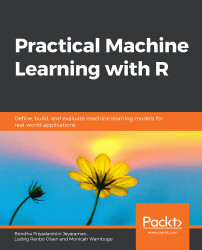•#### Practical Machine Learning with R#### Overview of this book

With huge amounts of data being generated every moment, businesses need applications that apply complex mathematical calculations to data repeatedly and at speed. With machine learning techniques and R, you can easily develop these kinds of applications in an efficient way. Practical Machine Learning with R begins by helping you grasp the basics of machine learning methods, while also highlighting how and why they work. You will understand how to get these algorithms to work in practice, rather than focusing on mathematical derivations. As you progress from one chapter to another, you will gain hands-on experience of building a machine learning solution in R. Next, using R packages such as rpart, random forest, and multiple imputation by chained equations (MICE), you will learn to implement algorithms including neural net classifier, decision trees, and linear and non-linear regression. As you progress through the book, you’ll delve into various machine learning techniques for both supervised and unsupervised learning approaches. In addition to this, you’ll gain insights into partitioning the datasets and mechanisms to evaluate the results from each model and be able to compare them. By the end of this book, you will have gained expertise in solving your business problems, starting by forming a good problem statement, selecting the most appropriate model to solve your problem, and then ensuring that you do not overtrain it.Free Chapter
An Introduction to Machine LearningData Cleaning and Pre-processingFeature EngineeringIntroduction to neuralnet and Evaluation MethodsLinear and Logistic Regression ModelsUnsupervised LearningAppendix## Regression

Regression models are used to predict the value of a dependent variable from a set of independent variables, and to inform us about the strengths and forms of the potential relationships between each independent variable and the dependent variable in our dataset. While we will only cover linear and logistic regression in this chapter, it is worth noting that there are more types of regression, such as Poisson regression, for predicting count variables, such as the number of tattoos a person has, and ordinal regression, for predicting ranked variables, such as questionnaire answers ("Really Bad", "Bad", "Decent", "Good", "Really Good"), where the difference between "Decent" and "Good" is not necessarily the same as between "Really bad" and "Bad".

Each of these regression models relies on a set of assumptions about the data. For instance, in order to meaningfully use and interpret a linear regression...